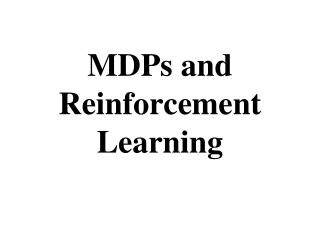DownloadDownload PresentationMDPs and Reinforcement Learning

# MDPs and Reinforcement Learning

Download Presentation## MDPs and Reinforcement Learning

- - - - - - - - - - - - - - - - - - - - - - - - - - - E N D - - - - - - - - - - - - - - - - - - - - - - - - - - -
##### Presentation Transcript

1. MDPs and Reinforcement Learning

2. Overview • MDPs • Reinforcement learning

3. Sequential decision problems • In an environment, find a sequence of actions in an uncertain environment that balance risks and rewards • Markov Decision Process (MDP): • In a fully observable environment we know initial state (S0) and state transitions T(Si, Ak, Sj) = probability of reaching Sj from Si when doing Ak • States have a reward associated with them R(Si) • We can define a policy π that selects an action to perform given a state, i.e., π(Si) • Applying a policy leads to a history of actions • Goal: find policy maximizing expected utility of history

4. 4x3 Grid World

5. 4x3 Grid World • Assume R(s) = -0.04 except where marked • Here’s an optimal policy

6. 4x3 Grid World Different default rewards produce different optimal policies life=pain, get out quick Life = struggle, go for +1, accept risk Life = good, avoid exits Life = ok, go for +1, minimize risk

7. Finite and infinite horizons • Finite Horizon • There’s a fixed time N when the game is over • U([s1…sn]) = U([s1…sn…sk]) • Find a policy that takes that into account • Infinite Horizon • Game goes on forever • The best policy for with a finite horizon can change over time: more complicated

8. Rewards • The utility of a sequence is usually additive • U([s0…s1]) = R(s0) + R(s1) + … R(sn) • But future rewards might be discounted by a factor γ • U([s0…s1]) = R(s0) + γ*R(s1) + γ2*R(s2)…+ γn*R(sn) • Using discounted rewards • Solves some technical difficulties with very long or infinite sequences and • Is psychologically realistic

9. Value Functions • The value of a state is the expected return starting from that state; depends on the agent’s policy: • The value of taking an action in a stateunder policy p is the expected return starting from that state, taking that action, and thereafter following p :

10. Bellman Equation for a Policy p The basic idea: So: Or, without the expectation operator:

11. Values for states in 4x3 world